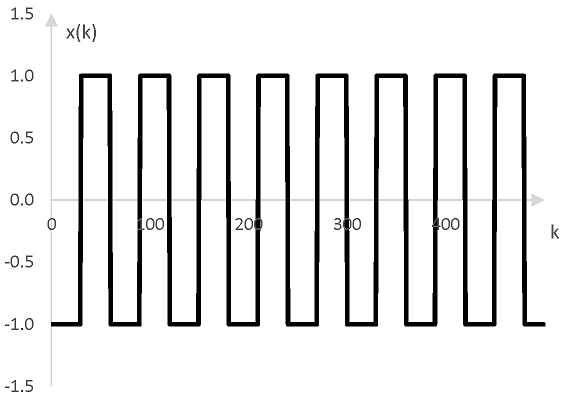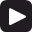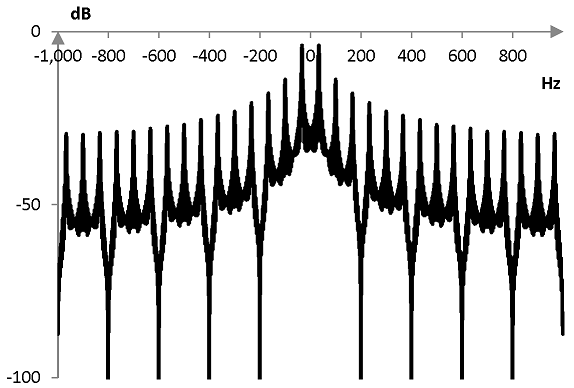# Square wave

A square wave is a periodic function that takes only two values – a minimum and a maximum – and alternates between these two values in equal intervals.

In discrete time, for example, if N is the period of the function, then the function

$$x(k)=\begin{cases} -1, k\, mod \, N \lt\frac{N}{2} \\ 1, k \, mod N \ge \frac{N}{2} \end{cases}$$

is a square wave. It alternates between -1 and 1. Its period is N samples. It takes each value for an interval equal to N/2 samples before switching to the other value.

Alternatively,

$$x(k)=sgn(sin(\frac{2 \pi\,k\,f}{f_s}))$$

since the sign function "sgn" is equal to 1 when its argument is positive and to -1 when its argument is negative. However, care must be taken where the argument is zero, as, as usually defined, sgn(0) = 0. Here, f is the desired square wave frequency and fs is the sampling frequency.

If, for example, N = 60, then the first function above will be as follows.The following is a square wave with the frequency of middle C.

Click Play to hear a square wave.## Using square waves

Analysis of the frequency content of square waves show that they can be thought of as the combination of many harmonics. Below, for example, is the discrete Fourier transform of 500 components of the example square wave above.

The harmonics in the square wave are all integer multiples of the frequency of the square wave. Note the evenly occurring notches in the magnitudes of frequencies present in the square wave.Because of these harmonics, square waves are often used in synthesizers. Square waves with the desired harmonics can be combined and the result filtered (e.g., through a low pass filter) to synthesize a sound resembling the sound of a specific instrument.

### Filtered HTML

• Freelinking helps you easily create HTML links. Links take the form of [[indicator:target|Title]]. By default (no indicator): Click to view a local node.